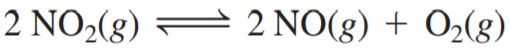×
Get Full Access to Chemistry: A Molecular Approach - 3 Edition - Chapter 14 - Problem 97e
Get Full Access to Chemistry: A Molecular Approach - 3 Edition - Chapter 14 - Problem 97e

×

# A sample of pure NO2 is heated to 337 °C, at whichISBN: 9780321809247 1

## Solution for problem 97E Chapter 14

Chemistry: A Molecular Approach | 3rd Edition

• Textbook Solutions
• 2901 Step-by-step solutions solved by professors and subject experts
• Get 24/7 help from StudySoup virtual teaching assistantsChemistry: A Molecular Approach | 3rd Edition

4 5 1 376 Reviews
20
1
Problem 97E

A sample of pure NO2 is heated to 337 °C, at which temperature it partially dissociates according to the equation:At equilibrium the density of the gas mixture is 0.520 g/L at 0.750 atm. Calculate Kc for the reaction.

Step-by-Step Solution:

Solution 97EStep 1The equilibrium reaction of NO, is as follows:Here. K is the equilibrium constant expressed in term of concentration.According to the data, the density of mixture of gases at equilibrium is 0.520 g/L at a pressure of0.750 atm.

Step 2 of 7

Step 3 of 7

##### ISBN: 9780321809247

Chemistry: A Molecular Approach was written by and is associated to the ISBN: 9780321809247. This textbook survival guide was created for the textbook: Chemistry: A Molecular Approach, edition: 3. The answer to “?A sample of pure NO2 is heated to 337 °C, at which temperature it partially dissociates according to the equation: At equilibrium the density of the gas mixture is 0.520 g/L at 0.750 atm. Calculate Kc for the reaction.” is broken down into a number of easy to follow steps, and 39 words. Since the solution to 97E from 14 chapter was answered, more than 680 students have viewed the full step-by-step answer. The full step-by-step solution to problem: 97E from chapter: 14 was answered by , our top Chemistry solution expert on 02/22/17, 04:35PM. This full solution covers the following key subjects: according, atm, calculate, Density, dissociates. This expansive textbook survival guide covers 82 chapters, and 9454 solutions.

## Discover and learn what students are asking

Calculus: Early Transcendental Functions : Product and Quotient Rules and Higher-Order Derivatives
?Using the Product Rule In Exercises 1–6, use the Product Rule to find the derivative of the function. \(g(x)=\left(x^{2}+3\right)\left(x^{2}-4 x\

Unlock Textbook Solution